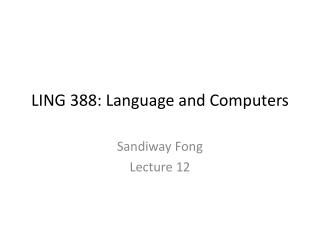Download PresentationLING 388: Language and ComputersLING 388: Language and Computers - PowerPoint PPT Presentation

Download PresentationLING 388: Language and Computers
An Image/Link below is provided (as is) to download presentation

Download Policy: Content on the Website is provided to you AS IS for your information and personal use and may not be sold / licensed / shared on other websites without getting consent from its author. While downloading, if for some reason you are not able to download a presentation, the publisher may have deleted the file from their server.

- - - - - - - - - - - - - - - - - - - - - - - - - - - E N D - - - - - - - - - - - - - - - - - - - - - - - - - - -
Presentation Transcript

1. LING 388: Language and Computers Sandiway Fong Lecture 12

2. Adminstrivia • Review: • Homework 4 on Recursion • Continue on with the left to right recursion grammar transformation: • Last Time: abstract grammar fragment • Today: PP adjunction to NP and VP

3. Homework 4 Review • Recursion type 1: • we can stack adjectives… • the bus • the big bus • the big red bus • the shiny big red bus • (cf. the big shiny red bus) • Recursive rule: • right recursive rule • nn --> a, nn.

4. Homework 4 Review Start with grammar.pl from the course webpage: • nn(nn(A,NN)) --> a(A), nn(NN). • a(jj(big)) --> [big]. • a(jj(shiny)) --> [shiny]. • a(jj(red)) --> [red]. • nn(nn(bus)) --> [bus]. sentence(s(X,Y)) --> np(X), vp(Y). pp(pp(X,Y)) --> in(X), np(Y). in(in(with)) --> [with]. np(np(X)) --> prp(X). np(np(np(X,Y),Z)) --> det(X), nn(Y), pp(Z). np(np(D,NN)) --> det(D), nn(NN). prp(prp(i)) --> [i]. prp(prp(me)) --> [me]. nn(nn(boy)) --> [boy]. nn(nn(telescope)) --> [telescope]. vp(vp(V,X)) --> verb(V), np(X). vp(vp(V,X,Y)) --> verb(V), np(X), pp(Y). verb(vbd(saw)) --> [saw]. det(dt(the)) --> [the]. det(dt(a)) --> [a].

5. Homework 4 Review • Tree representation:

6. Homework 4 Review • Tree representation:

7. Homework 4 Review • Tree representation:

8. Homework 4 Review • Tree representation:

9. Homework 4 review • Recursion is a property of natural language • simple iteration: … big shiny red bus • Another kind of recursion • Some verbs can select for clauses (as well as object NPs), e.g. notice • I noticed that John noticed that Mary noticed the big red bus • [S I noticed [SBAR that [S John noticed [SBAR that [S Mary noticed the big red bus]]]]] Idea: some verbs can select for sentences introduced by the complementizerthat

10. Homework 4 review • Example: • Mary noticed the big red bus verb(vbd(noticed)) --> [noticed]. np(np(X)) --> proper_noun(X). proper_noun(nnp(mary)) --> [mary].

11. Homework 4 review • Example: • John noticed that Mary noticed the big red bus (IN that) vp(vp(V,SBAR)) --> verb(V), sbar(SBAR). sbar(sbar(C,S)) --> c(C), sentence(S). c(c(that)) --> [that]. proper_noun(nnp(john)) --> [john].

12. Homework 4 review • Example: • I noticed that John noticed that Mary noticed the big red bus

13. Homework 4 review • Example: • I noticed that John noticed that Mary noticed the big red bus

14. Today’s Topic • Exercise: • Convert left recursive natural language grammar rules into right recursive grammar rules

15. Step 1 • Recipe: • Let x be the nonterminal with the left recursion. • Let z be the terminal (or nonterminal) sequence that x also expands to • Let y be the terminal (and/or nonterminal) sequence after the left recursive call • Abstract example from last time: • x --> x, [y]. • x --> [z].

16. Step 1 x --> x, [y]. x --> [z]. • Part 1: • identify x, y, and z in the following rules: • np --> dt, nn. • np --> np, pp. • identify x, y, and z in the following rules: • vp --> vbd, np. • vp --> vp, pp.

17. Step 2 • Transformation: • x --> x, [y]. • x --> [z]. into • x --> [z], v. • v --> [y], v. • v --> [y]. • x --> [z]. • Step 2: • apply transformation to: • np --> dt, nn. • np --> np, pp. also to: • vp --> vbd, np. • vp --> vp, pp.

18. Step 3 • Step 3: • modify the grammar fragments from step 2 into grammars that compute parse trees • test your grammar fragments on VPs like saw a boy with a telescope • vp(Parse,[saw, a, boy, with, a, telescope], []). • Abstract example: • x --> [z], v. • v --> [y], v. • v --> [y]. • x --> [z]. modified version: • x(x(z,V)) --> [z], v(V). • v(v(y,V)) --> [y], v(V). • v(v(y)) --> [y]. • x(x(z)) --> [z].

19. Comparison • “Quick Fix” grammar from last time: • Result:

20. Step 4 • Step 4: • Compare the transformed grammar parses with those obtained using the “Quick Fix” grammar on the NP • a boy with a telescope with a limp • number of parses? • attachment of the PPs?